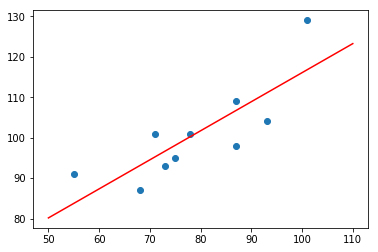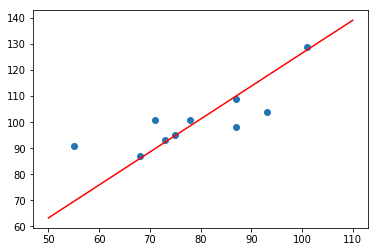## 线性回归最小二乘法与梯度下降法数学推导及 Python 实现

2116 字 · 3353 阅读 · 2018 年 10 月 10 日### 最小二乘法代数求解推导### 最小二乘法代数求解实现

x = [55, 71, 68, 87, 101, 87, 75, 78, 93, 73]
y = [91, 101, 87, 109, 129, 98, 95, 101, 104, 93]


def ols_algebra(x, y):
n = len(x)
w1 = (n*sum(x*y) - sum(x)*sum(y))/(n*sum(x*x) - sum(x)*sum(x))
w0 = (sum(x*x)*sum(y) - sum(x)*sum(x*y))/(n*sum(x*x)-sum(x)*sum(x))
return w0, w1


import numpy as np

ols_algebra(np.array(x), np.array(y))

&gt;&gt;&gt; (44.25604341391219, 0.7175629008386778)


### 最小二乘法矩阵求解推导### 最小二乘法矩阵求解实现

def ols_matrix(x, y):
w = (x.T * x).I * x.T * y
return w


x = np.array(x).reshape(len(x), 1)
x = np.concatenate((np.ones_like(x), x), axis=1)
x = np.matrix(x)

y = np.array(y).reshape(len(y), 1)
y = np.matrix(y)

ols_matrix(x, y)

&gt;&gt;&gt; matrix([[44.25604341], [ 0.7175629 ]])


$$y = 44.256 + 0.718 * x$$

import matplotlib.pyplot as plt

plt.scatter(x, y)
plt.plot(np.array([50, 110]), np.array([50, 110]) * 0.718 + 44.256, 'r')### 梯度下降法求解数学推导### 梯度下降法求解代码实现

def gradient_descent(x, y, lr, num_iter):
w1 = 0  # 初始参数为 0
w0 = 0  # 初始参数为 0

for i in range(num_iter):  # 梯度下降迭代
# 计算近似值
y_hat = (w1 * x) + w0
# 计算参数对应梯度
w1_gradient = -(2/len(x)) * sum(x * (y - y_hat))
w0_gradient = -(2/len(x)) * sum(y - y_hat)
# 根据梯度更新参数

return w1, w0


w1, w0 = gradient_descent(np.array(x), np.array(y), lr=0.00001, num_iter=100)
w1, w0

&gt;&gt;&gt; (1.264673601681181, 0.017892580143733863)


plt.scatter(x, y)
plt.plot(np.array([50, 110]), np.array([50, 110]) * w1 + w0, 'r')### 小结•